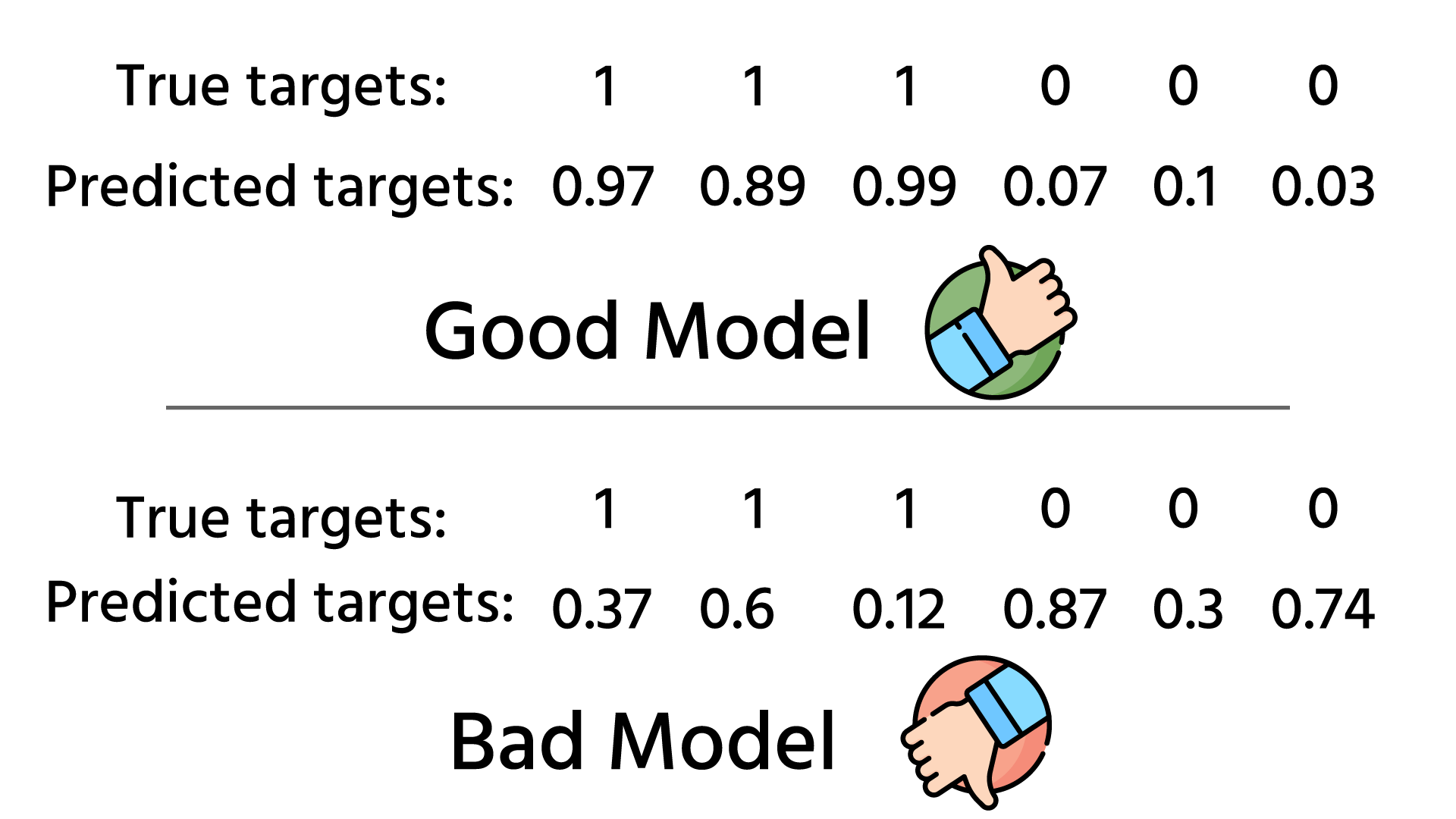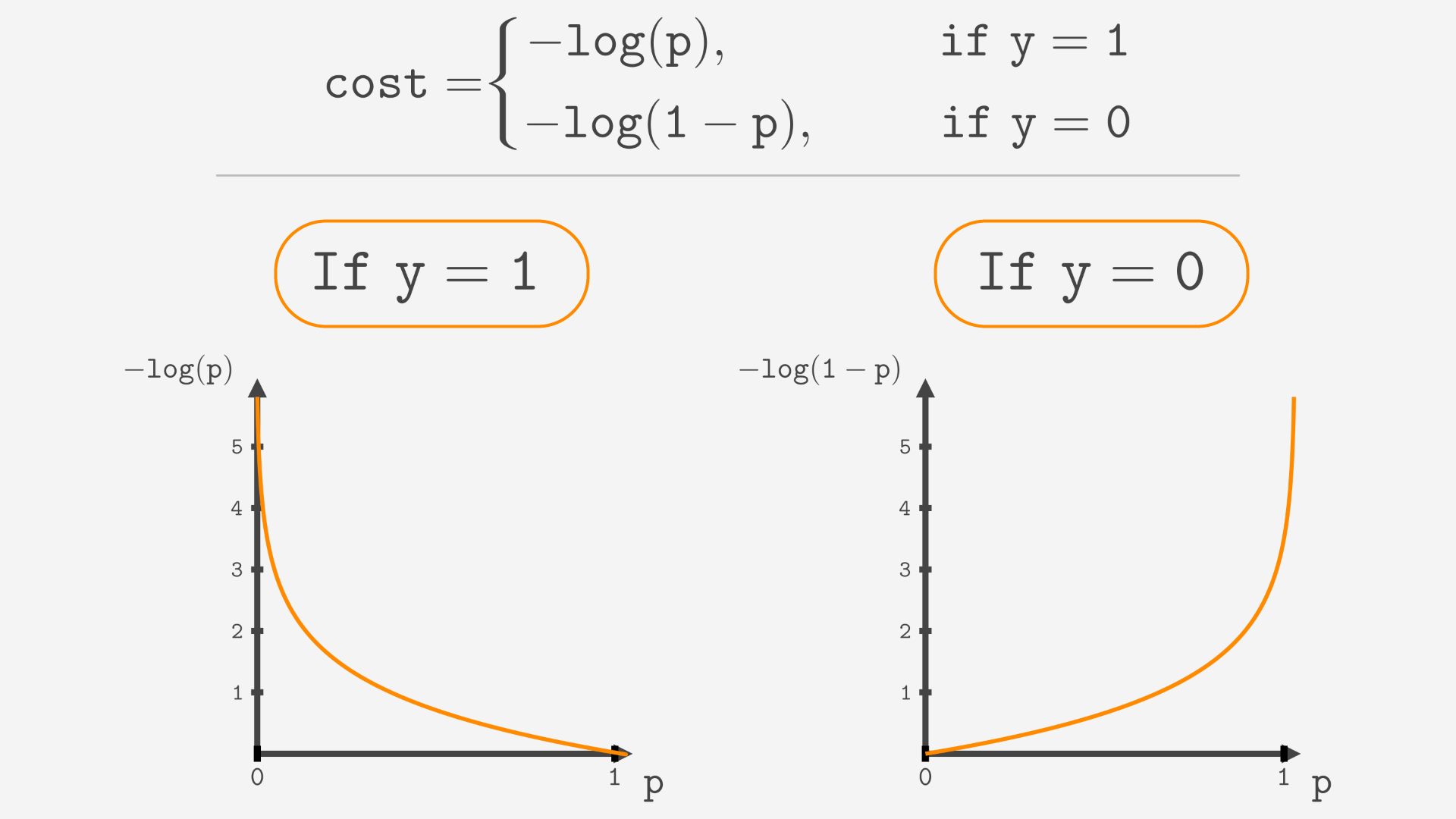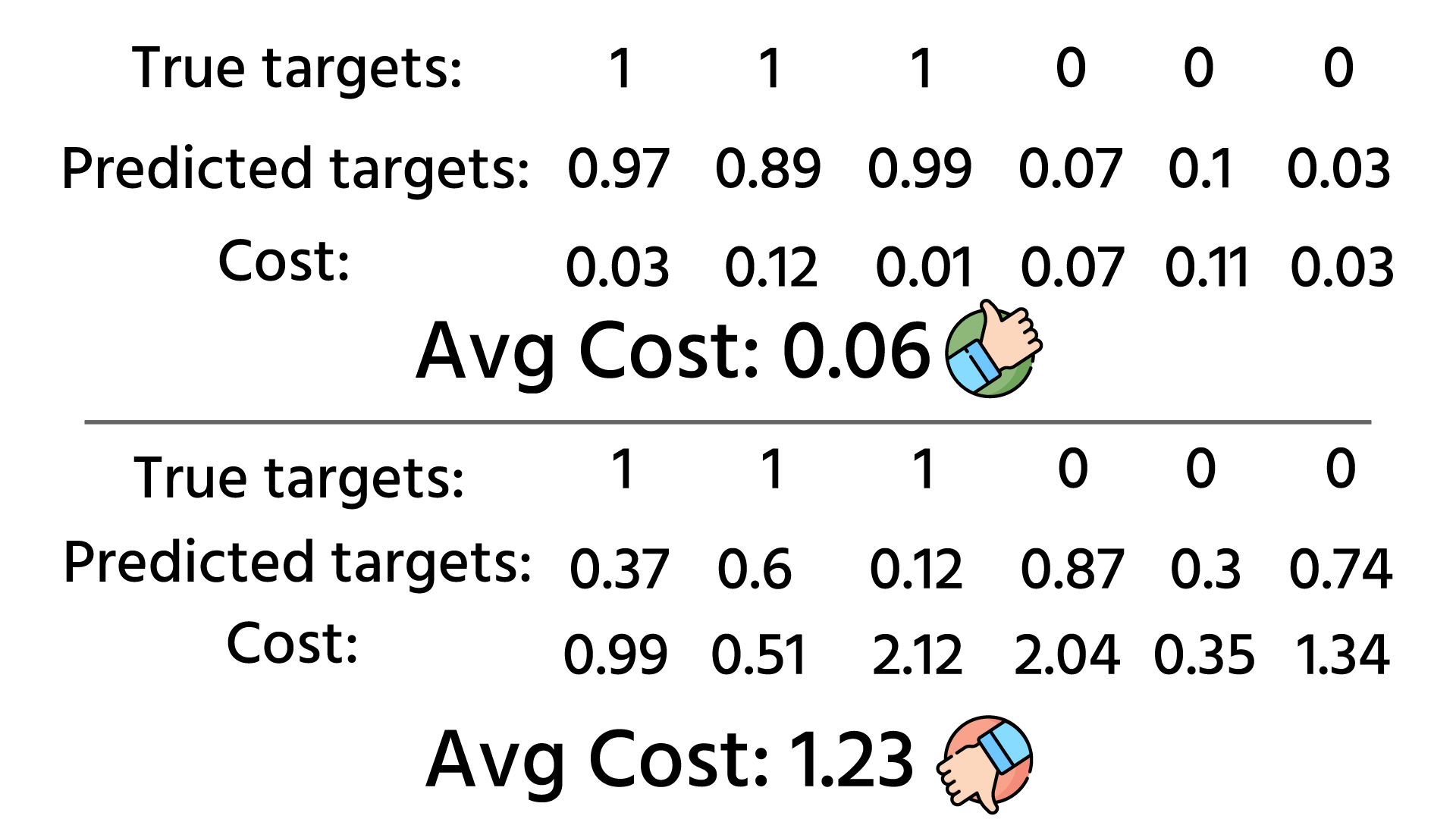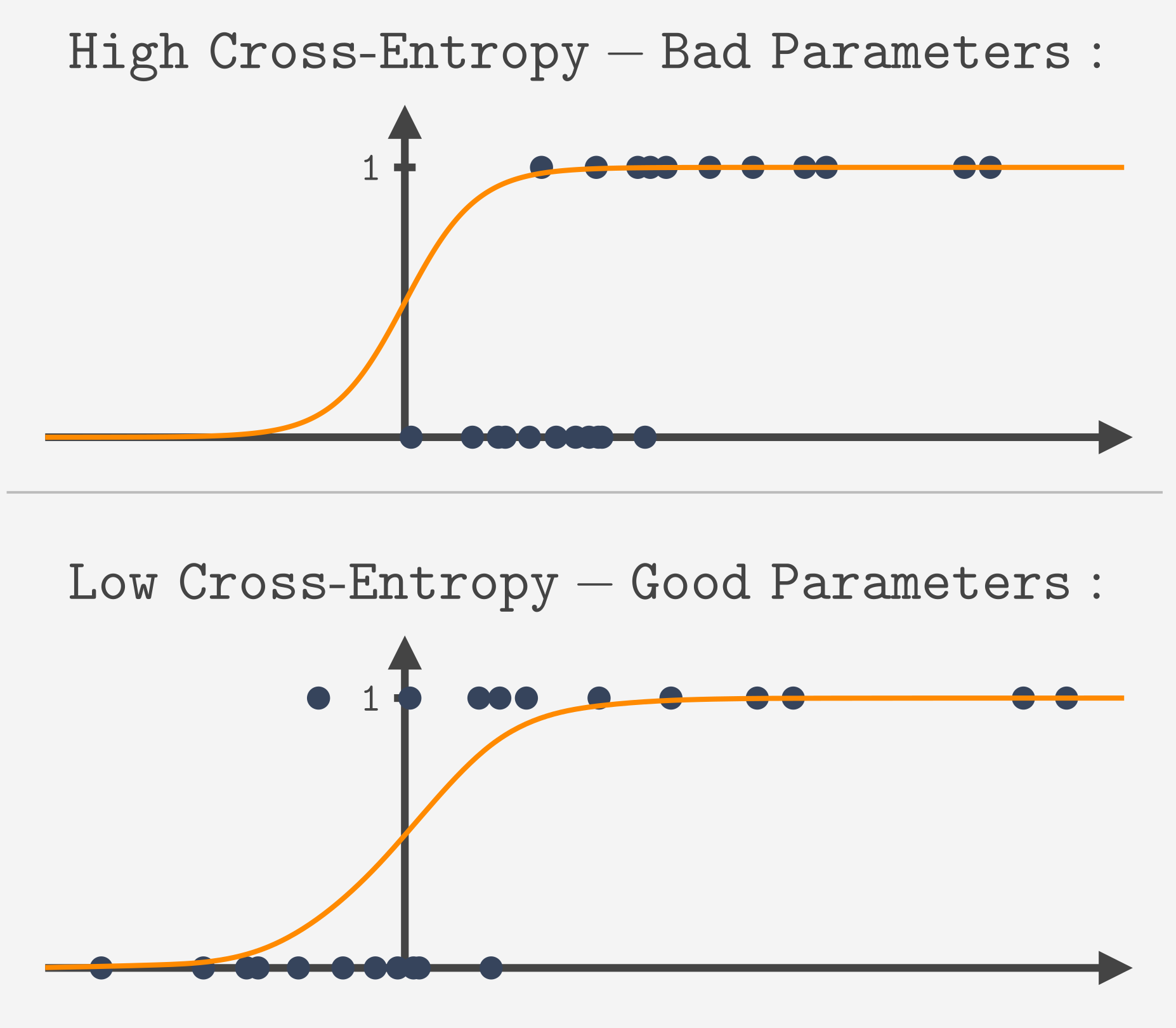Course Content

Classification with Python

##Finding The Parameters

Logistic Regression only requires from computer to learn the best parameters β. For that, we need to define what "best parameters" means. Let's recall how the model works, it predicts the p - probability of belonging to class 1.Obviously, the model with good parameters is the one predicting high (close to 1) p for instances that are actually of class 1 and low (close to 0) p for instances with the actual class 0.To measure how bad or how good the model is, we use a cost function. In linear regression, we used SSR as a cost function. This time, a different function is used:Here p is the probability of belonging to class 1, predicted by the model, and y is the actual target value.

This function not only penalizes incorrect predictions but also accounts for how confident the model was in its prediction.
As you can see from the image above, if the value of p is close to y (actual target), then the cost function is relatively small. It means that the model confidently chose the correct class.
But if the prediction is incorrect, the cost function grows exponentially as the model's confidence in the wrong class increases.We calculate the cost function for each training instance and take the average. This cost function is called Cross-Entropy Loss. So Logistic Regression just finds the parameters β that minimize Cross-Entropy Loss.Everything was clear?

Section 2. Chapter 2